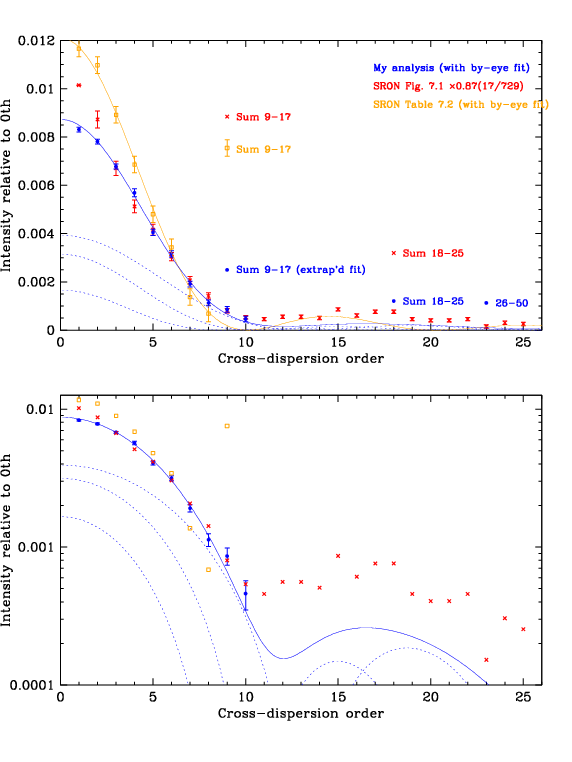LETG Cross-Dispersion Order Intensities

## Appendix A: LETG Cross-Dispersion Order Intensities

### Previous Work

The only previous documented analysis is in SRON's "AXAF-LETGS calibration report, Draft Final" (Nov 15 1998), Section 7.1 "Fine support line" on pages 79-82. Their Table 7.2 lists "Quick look" results assuming bar/period ratios of 0.50 for the main grating and 0.10 for fine support structure. No other information is provided about this model. In the figure below we plot those numbers in orange with significant digit errorbars and a sinc2 function, appropriate for ideal gratings, of the form I(m) = 0.0121((sin(0.31m))/0.31m)2 where the intensity I is normalized to the 0th cross-dispersed order and m is the cross-dispersion order.

The SRON report also plots relative intensities measured at the XRCF in Figure 7.1, using Al-K line emission (with some continuum). O-K measurements were also made but were of lower quality. A family of sinc2 functions are also plotted in that figure, none of which match the measured points very well. In particular, the model curves have minima around m=10 with zero intensity but the measured intensities never reach zero. Intensities in that Figure are normalized to m=1. After reading their values from that Figure we rescaled them to 0th order intensity using numbers from Table 7.2 (I(1)/I(0)=17/729) and then multipled by another factor of 0.87 to give better agreement with our measurements. Those results are plotted in the figure below in red.

### This Work

Our measurements are plotted in blue. As noted above, the sinc2 functions in ideal grating equations have minima at zero intensity, unlike the measured values. We used a combination of three functions to fit our measurements:

```I(m) = 0.19 × 0.00873((sin(0.36m))/0.36m)2
+ 0.36 × 0.00873((sin(0.30m))/0.30m)2
+ 0.45 × 0.00873((sin(0.24m))/0.24m)2
```
The different sinc arguments reflect different bar/period ratios of the fine support structure, but such a large parameter variation is unphysical. The cause of the significant disagreement between observed intensities and single-grating-model predictions remains unclear.Fig. A1. Model and measured cross-dispersion-order intensities from the SRON calibration report (orange and red) and the current work (blue). Our model is a sum (solid blue line) of three sinc2 functions, shown separately (dotted lines).

### Discussion

The SRON Table 7.2 results are only shown to emphasize that the "Quick Look" model is idealized and not appropriate for the real LETG. The Figure 7.1 results are based on measurements but we believe that SRON's analysis, which was a minor part of a much larger LETG calibration effort completed within a relatively short time after XRCF calibration, suffers from errors in background determination, particularly for m=1 and 2 because of the m=0 LSF wings. A reanalysis of XRCF data would shed light on these discrepancies but we are confident that our analysis is significantly more accurate than previous work and the net effect of remaining errors on LETG/HRC-S calibration is negligible.